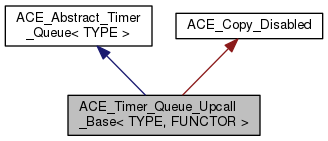ACE  6.3.4
ACE_Timer_Queue_Upcall_Base< TYPE, FUNCTOR > Class Template Reference

`#include <Timer_Queue_T.h>`

Inheritance diagram for ACE_Timer_Queue_Upcall_Base< TYPE, FUNCTOR >:[legend]
Collaboration diagram for ACE_Timer_Queue_Upcall_Base< TYPE, FUNCTOR >:[legend]

## Public Member Functions

ACE_Timer_Queue_Upcall_Base (FUNCTOR *upcall_functor=0)

virtual ~ACE_Timer_Queue_Upcall_Base (void)
Destructor. More...

FUNCTOR & upcall_functor (void)
Accessor to the upcall functor. More...Public Member Functions inherited from ACE_Abstract_Timer_Queue< TYPE >
virtual ~ACE_Abstract_Timer_Queue (void)=0
Destructor. More...

virtual bool is_empty (void) const =0
True if queue is empty, else false. More...

virtual const ACE_Time_Valueearliest_time (void) const =0

virtual long schedule (const TYPE &type, const void *act, const ACE_Time_Value &future_time, const ACE_Time_Value &interval=ACE_Time_Value::zero)=0

virtual int expire (const ACE_Time_Value &current_time)=0

virtual int expire (void)=0

virtual int expire_single (ACE_Command_Base &pre_dispatch_command)=0

virtual int reset_interval (long timer_id, const ACE_Time_Value &interval)=0

virtual int cancel (const TYPE &type, int dont_call_handle_close=1)=0

virtual int cancel (long timer_id, const void **act=0, int dont_call_handle_close=1)=0

virtual int close (void)=0

virtual ACE_Time_Value gettimeofday (void)=0

virtual void gettimeofday (ACE_Time_Value(*gettimeofday)(void))=0

virtual ACE_Time_Valuecalculate_timeout (ACE_Time_Value *max)=0

virtual ACE_Time_Valuecalculate_timeout (ACE_Time_Value *max, ACE_Time_Value *the_timeout)=0

virtual ACE_Time_Value current_time ()=0

virtual ITERATORiter (void)=0
Returns a pointer to this ACE_Timer_Queue's iterator. More...

virtual ACE_Timer_Node_T< TYPE > * remove_first (void)=0
Removes the earliest node from the queue and returns it. More...

virtual ACE_Timer_Node_T< TYPE > * get_first (void)=0
Reads the earliest node from the queue and returns it. More...

virtual void dump (void) const =0
Dump the state of a object. More...

## Protected Attributes

FUNCTOR * upcall_functor_
Upcall functor. More...

bool const delete_upcall_functor_
To delete or not to delete is the question? More...Public Types inherited from ACE_Abstract_Timer_Queue< TYPE >
typedef ACE_Timer_Queue_Iterator_T< TYPE > ITERATOR
Type of Iterator. More...Private Member Functions inherited from ACE_Copy_Disabled
ACE_Copy_Disabled (void)
Default constructor. More...

## Detailed Description

### template<typename TYPE, typename FUNCTOR> class ACE_Timer_Queue_Upcall_Base< TYPE, FUNCTOR >

Common base class for all timer queues with the same time source policy. This is really motivated by a single use-case in the code, namely the ACE_Proactor needs to set a backpointer in the upcall functor.

## Constructor & Destructor Documentation

template<class TYPE , class FUNCTOR >
 ACE_Timer_Queue_Upcall_Base< TYPE, FUNCTOR >::ACE_Timer_Queue_Upcall_Base ( FUNCTOR * upcall_functor = `0` )
inlineexplicit
template<class TYPE , class FUNCTOR >
 ACE_Timer_Queue_Upcall_Base< TYPE, FUNCTOR >::~ACE_Timer_Queue_Upcall_Base ( void )
inlinevirtual

Destructor.

## Member Function Documentation

template<class TYPE , class FUNCTOR >
 FUNCTOR & ACE_Timer_Queue_Upcall_Base< TYPE, FUNCTOR >::upcall_functor ( void )
inline

Accessor to the upcall functor.

## Member Data Documentation

template<typename TYPE , typename FUNCTOR >
 bool const ACE_Timer_Queue_Upcall_Base< TYPE, FUNCTOR >::delete_upcall_functor_
protected

To delete or not to delete is the question?

template<typename TYPE , typename FUNCTOR >
 FUNCTOR* ACE_Timer_Queue_Upcall_Base< TYPE, FUNCTOR >::upcall_functor_
protected

Upcall functor.

The documentation for this class was generated from the following files: